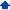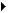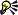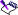### Calculating formulas in tables

WordPerfect lets you perform spreadsheet calculations on table data. For example, you can calculate formulas, add values in a table quickly, and ignore a cell during calculations.

Formulas are calculated automatically each time you move the insertion point or insert a formula using the Table formula toolbar. You can disable automatic calculation.

You can also fill in cells with incrementing patterns and dates. A pattern of values must increment or decrement based on addition or subtraction. You cannot fill in a pattern of values that increment or decrement based on multiplication or division.

You can get more information about table formula errors, including a brief explanation of any ?? and ERR error symbols that display in a table as you calculate formulas.

#### To calculate a formula in a table1 Click in a table.
 2 Click TableCalculate.
 3 Enable one of the following options:
 • Calculate table — calculates the table or floating cell in which the insertion point is positioned
 • Calculate tables in document — calculates all tables and floating cells in the document• You can disable automatic calculation by enabling the Off option.

#### To add values in a table quickly1 Click the table cell below or to the right of the cells whose total you want to calculate.
 2 Click TableQuickSum.• The calculated sum overwrites any values, formulas, or text in the cell you click.• You can also add values by clicking the QuickSum button on the Table formula toolbar. To display the Table formula toolbar, click TableFormula toolbar.

#### To ignore a table cell during calculations1 Select a cell, and click TableFormat.
 2 Click the Cell tab.
 3 Enable the Ignore cell when calculating check box.

#### To fill a table with incremental numbers or dates1 Select the cells that contain the incremental or decremental pattern of val ues as well as the cells in which you want to continue the pattern.
 2 Click TableQuickFill.• You can use Roman numerals, days of the week, months, or quarters, even if only one cell is used to establish the pattern. To repeat incremental or decremental numbers, however, you must select at least two cells with values to establish a pattern.• You can also fill a table with incrementing numbers or dates by clicking the QuickFill button on the Table formula toolbar. To display the Table formula toolbar, click TableFormula toolbar.